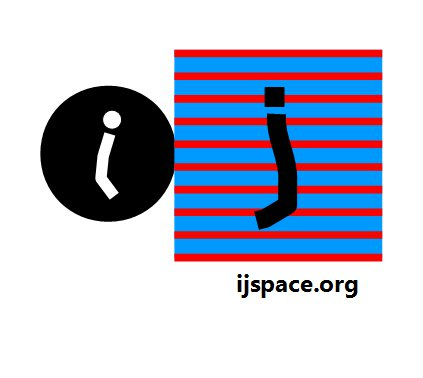Home Summary: The interaction between the base states and the energy requirements are discussed.The interaction between the base states follows the properties, 1. The information loss is continuous in j-space i.e. eventually all the measurement states will convert into the low-energy state <↑↑↑>, and 2. The macroscopic observer makes measurement by applying a minimal measurement force (≈ 0j) and hence only the low-energy measurement states are considered as the final structures. Let us consider a hypothetical example.  The objective is the measurement of  <↓↓↓> state by a macroscopic observer, and hence we describe the propagation of <↓↓↓> state into <↑↑↑'> state.   <↓↓↓> (I) + <↑↓↓> (II) → <↑↓↓>(I')                       (ia)              <↑↓↓> (I') + <↑↑↓> (III) → <↑↑↓>(II')                        (ib)              <↑↑↓> (II') + <↑↑↑> (IV) → <↑↑↑>'(III')                   (ic)              In above set of equations (I, II, III, IV) are base states and (I', II', III') are product states.       Each state can be considered as an observer, hence <↑↓↓>-observer has less capability than <↓↓↓>-observer.      Therefore the <↑↓↓>-observer can not measure <↓↓↓> state completely, and as a result a symmetry is assumed and the product state <↑↓↓> is created.       This new product state has much lower capability than the parent states.  It interacts with another base state III and another symmetry is assumed and state-II' is created.        The measurements made by the macroscopic observer are bound by entropy considerations.  The macroscopic observer also has difficulty measuring <↓> states without additional energy input.  For macroscopic observer the <↑↑↑> state is used as the measurement tool.       The <↑↑↑> state, if not given adequate energy, can not measure successfully state-II' and as a result <↑↑↑>' with altered dynamical property is created whose analogue in (t, x, y, z) space is a state with changed momentum.        The equation set (i) is not the only way to measure <↓↓↓> state.  Alternatively we can consider the following sequence: <↓↓↓> (I) + <↓↑↓> (II) → <↓↑↓> (I')        (2a)         <↓↑↓> (I') + <↑↑↑> (III) → <↑↑↑>' (II')       (2b)               However we note that in order to measure state-I' properly in eqn. 2b, two arrows need to be flipped.   Compare this to eqn. 1c which requires only one arrow to be flipped.  Hence the energy required to make a measurement of type 2b, the state <↑↑↑> has to be given lot more energy than for that of type 1c.       It is fairly obvious that the reaction of the type <↓↓↓> + <↑↑↑>  (i.e. one step reaction), is very difficult to achieve as the energy input required is extremely high.  Even if reasonably high energy condition is achieved (e.g. LHC), at best the macroscopic observer can expect, <↓↓↓> + <↑↑↑> = <↑↑↑>'                        (iii)                 where the dynamical characteristics of <↑↑↑> state is altered into <↑↑↑>' (an unstable state) and some partial inferences can be drawn from residual traces of the intermediate states, which again are dependent on the measurement conditions.  A complete and precise characterization of <↓↓↓> state, is unlikely using <↑↑↑> state.      A better alternative will be to use (<↓↑↑>, <↑↓↑>, <↑↑↓>) states.  Further the application of (<↓↑↓>, <↓↓↑>, <↑↓↓>) states may be problematic for ObsM, as these states will be difficult to create as each of them have two downward arrows.      We also note that <↑↑↑> state represents the measurement state used by macro-observer, rather than <↑↑↑> state. The <↑↑↑> state is a purely dynamic state and hence can not be measured accurately in j-space.        We can appreciate the problem of measuring <↓↓↓> primarily because the three <↓> states are being measured simultaneously.  And it really does not provide us with complete information about j-space.       A much more efficient alternative will be to improve the resolution of the measurements so that the requirement of measurements for all three <↓> states simultaneously, is removed i.e. a macroscopic observer should be able to measure (<↕>, <↕>, <↕>)  states individually or make measurements with capability equivalent to Obsj pair.  The results obtained in this case will be much more accurate and energy requirement will be within observer's capability.  It is advisable to perform such measurements in space where the effect of gravity is minimized.        The examples presented above are only hypothetical constructs.